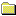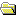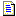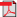Products Download Events Support Videos All Product Families ARM7, ARM9, and Cortex-M3 Products C16x, XC16x, and ST10 Products C251 and 80C251 Products Cx51 and 8051 Products Modified Anytime In the Last 7 Days In the Last 14 Days In the Last 30 Days In the Last 90 Days In the Last 6 Months

Technical Support

On-Line Manuals

Libraries and Floating Point Support GuidePrefaceThe ARM C and C++ LibrariesThe ARM C Micro-libraryFloating-point SupportAbout floating-point supportThe software floating-point library, fplibCalling fplib routinesfplib arithmetic on numbers in a particular formatfplib conversions between floats, long longs, doubfplib comparisons between floats and doublesfplib C99 functionsControlling the ARM floating-point environmentFloating-point functions for compatibility with MiC99-compatible functions for controlling the ARM fC99 rounding mode and floating-point exception macException flag handlingFunctions for handling rounding modesFunctions for saving and restoring the whole floatFunctions for temporarily disabling exceptionsARM floating-point compiler extensions to the C99Writing a custom exception trap handlerExample of a custom exception handlerException trap handling by signalsmathlib double and single-precision floating-pointIEEE 754 arithmeticBasic data types for IEEE 754 arithmeticSingle precision data type for IEEE 754 arithmeticDouble precision data type for IEEE 754 arithmeticSample single precision floating-point values forSample double precision floating-point values forIEEE 754 arithmetic and roundingExceptions arising from IEEE 754 floating-point arException types recognized by the ARM floating-poiUsing the Vector Floating-Point (VFP) support librThe C and C++ Library Functions referenceFloating-point Support Functions Reference

# <!-- var kmNextPage = "armlib_chr1358938950007.htm"; var kmPrevPage = "armlib_chr1358938947199.htm"; var kmNextPageTitle = "Double precision data type for IEEE 754 arithmetic"; var kmPrevPageTitle = "Basic data types for IEEE 754 arithmetic"; var kmBreadCrumbs = "<a href=\"default.htm\">Home</a> &raquo; <a href=\"armlib_chr1358938940724.htm\">Floating-point Support</a> &raquo; <a href=\"armlib_chr1358938946887.htm\">IEEE 754 arithmetic</a> &raquo; Single precision data type for IEEE 754 arithmetic"; kmSetupPaging (); kmNavButtons(); //--> Single precision data type for IEEE 754 arithmetic

### 3.5.2 Single precision data type for IEEE 754 arithmetic

A `float` value is 32 bits wide.

The structure is:
Figure 3-1 IEEE 754 single-precision floating-point formatThe `S` field gives the sign of the number. It is 0 for positive, or 1 for negative.
The `Exp` field gives the exponent of the number, as a power of two. It is biased by `0x7F` (127), so that very small numbers have exponents near zero and very large numbers have exponents near `0xFF` (255).
For example:
• If `Exp` = `0x7D` (125), the number is between 0.25 and 0.5 (not including 0.5).
• If `Exp` = `0x7E` (126), the number is between 0.5 and 1.0 (not including 1.0).
• If `Exp` = `0x7F` (127), the number is between 1.0 and 2.0 (not including 2.0).
• If `Exp` = `0x80` (128), the number is between 2.0 and 4.0 (not including 4.0).
• If `Exp` = `0x81` (129), the number is between 4.0 and 8.0 (not including 8.0).
The `Frac` field gives the fractional part of the number. It usually has an implicit 1 bit on the front that is not stored to save space.
For example, if `Exp` is `0x7F`:
• If `Frac` = `00000000000000000000000` (binary), the number is 1.0.
• If `Frac` = `10000000000000000000000` (binary), the number is 1.5.
• If `Frac` = `01000000000000000000000` (binary), the number is 1.25.
• If `Frac` = `11000000000000000000000` (binary), the number is 1.75.
In general, the numeric value of a bit pattern in this format is given by the formula:
(–1)S * 2(Exp–0x7F) * (1 + Frac * 2–23)
Numbers stored in this form are called normalized numbers.
The maximum and minimum exponent values, 0 and 255, are special cases. Exponent 255 can represent infinity and store Not a Number (NaN) values. Infinity can occur as a result of dividing by zero, or as a result of computing a value that is too large to store in this format. NaN values are used for special purposes. Infinity is stored by setting Exp to 255 and Frac to all zeros. If Exp is 255 and Frac is nonzero, the bit pattern represents a NaN.
Exponent 0 can represent very small numbers in a special way. If `Exp` is zero, then the `Frac` field has no implicit 1 on the front. This means that the format can store 0.0, by setting both `Exp` and `Frac` to all 0 bits. It also means that numbers that are too small to store using `Exp >= 1` are stored with less precision than the ordinary 23 bits. These are called denormals.
 Non-ConfidentialPDF version ARM DUI0378H Copyright © 2007, 2008, 2011, 2012, 2014-2016 ARM. All rights reserved.##### Important information

This site uses cookies to store information on your computer. By continuing to use our site, you consent to our cookies.